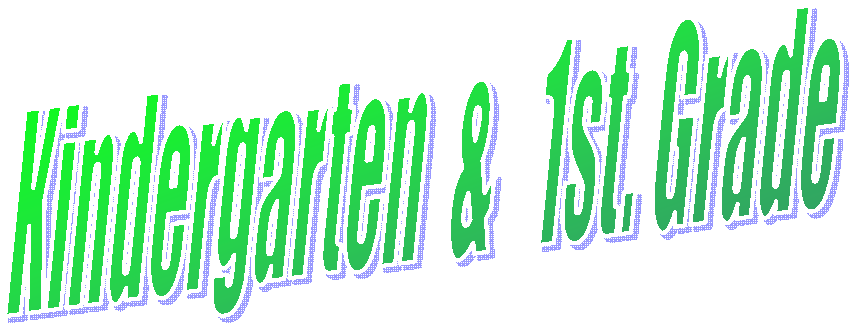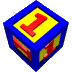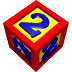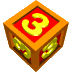Kindergarten & 1st. Grade Video Lessons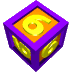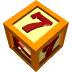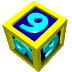KINDERGARTEN ASSESSMENTS (PAGE 1)   (PAGE 2)

 Shape Logic Problems Reading Links Addition & Subtraction Coded Message Power Point Spelling Words WRITING SKILLS STORIES New method of blending technology in your lessons.  DECK TOYS Lessons on words  and numbers Dick and Jane Stories Lesson on Mindful Writing Math Review with Videos Websites Using Coordinates for Drawing and Math
Lessons for the first month or two of Kindergarten.  You determine how long.

This site will introduce new concepts that are differentiated for the first part of Kindergarten.
Math Lessons   K 1. Lesson Word problems counting up to 5  4 Minutes long
Word Problems   K 2. Lesson Number problems counting up to 6  4 Minutes long
K 3. Lesson Problems counting up to 8 using shapes   4 Minutes long
K 4. Lesson Problems fishing and counting   4 Minutes long
Number Sense   K 1. Lesson Counting practice writing numbers from 1 to 10
K 2. Lesson Determine which numbers is the largest one or smaller one
K 3. Lesson Determine the order of numbers from smallest to largest
1 4. Lesson Counting practice writing numbers from 1 to 120 2:41
1 5. Lesson Counting practice writing numbers from 1 to 120 6:30
Math word problems  Operations and Algebraic Thinking 1.OA 1 6. Lesson You will find 15 pages of math problems that you may use for your students.  I have saved the lesson in a RTF format so you may differentiate the lesson to your liking.

FUN  EDUCATIONAL  GAMES  LISTED  BELOW

TEACHING TOOLS Color  Count Numbers Make An Animation Story Maker
Color by Number Coloring Autumn Leaves Spot Color the Letter Count the numbers
All small numbers
Add - Subtract - Multiply - Divide
Creating Animation
Give Teddy Color Eggs Counting Toys Spider Alphabet Story Place Marvin Makes Music Story Book Lilly the wild Cat music Story Book

K - 1 1. Lesson -1 Addition using a number line from 0  - 10
K - 1 2. Lesson -2 Addition using a number line from 0 -  12
K - 1 3. Lesson -3 Addition using a number line from 0  - 20 Columns
K - 1 4. Lesson -4 Addition using a number line from 0 -  20  Columns

New Method of Teaching Math
Deck Toys

NB = New Background

1. Counting Carrots 16. Counting Dominos New lesson added
2. Counting Unicorns 1-10 17. Christmas Jigsaw Puzzles
3. Colors and Numbers 18. Learning the Alphabet, Numbers,  and logic NB
4. Counting Spider-man 19. Addition Logic 1  It's not this one
Directions:  Students click on the first sign that wiggles.  They then go to the next object that wiggles ( FLASH CARDS) Must complete each game before they can move on. 5. Counting Balloons 20. Learning Alphabet and Numbers - 2
6. Counting Bambi - 2 NB 21. NB
7. Counting 1 digit numbers 22. Adding up to 10
9. Counting 1 digit numbers 24.Ten Frame Addition
11. Addition and Place Value 26. Matching Number and words 1 - 5
12. Counting 1 - 10  Apples 27. Addition  1 - 10 True or False - 1
13. Missing Numbers Ten Frame 28. Addition and Subtraction True and False
15. Counting with Fingers 3) Review Colors and Shapes
Balancing Numbers
Great Interactive Lessons for Math

TRY THE LINKS BELOW FOR FUN AND EDUCATIONAL

Different Disguise Visual Memory Xylophone Hidden Picture Farm Hidden Picture  Beach Hidden Picture Bus Stop
Sticker  Cartoon Animation Activity Fruit Fall Picture Game (Graph) Missing Addend Baseball Counting Game Road Sign Maker Hidden Picture Dragon
Math Test - Addition - Subtraction -  Multiplication- Division Shapes   Falling Monkeys fly Pop the Balloons Interactive Clock Telling Time 1. Super Hero   Pattern
2. Super Hero Shape Pattern

The Train and Words

3. Super Hero  Number Pattern
Outstanding on line interactive lessons in many areas

K - 2 Interactive lessons    Flash is required

Compare and Order Numbers
Requires Flash
) for Language Arts - Math - Logic   Outstanding Website For All Areas of Learning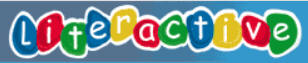Starfall

Lil Fingers

PEG & CAT
STORIES

Working with Pictures and Words
Interactive
Very Good    Website

Story-time

Stories

DAYS OF THE WEEK

Audio Concentration      Website

FOR Kindergarten   QR CODES  Lessons in -- Math, Language Arts, Science, Social Studies
FOR 1st Grade   QR CODES  Lessons in -- Math, Language Arts, Science, Social Studies

COUNTING BLOCK IMAGES

K - 1 AA. LESSON AA Count the number of blocks in each unit that are colored
K - 1 1. LESSON 1 Count the number of blocks in each unit and circle the answer
K - 1 2. LESSON 2 Count the number of blocks in each unit and circle the answer
K - 1 3. LESSON 3 Count the number of blocks in each unit and circle the answer

Balancing Numbers
Great Interactive Lessons for Math

PUZZLES FOR NUMBERS AND ALPHA
Cut each puzzle strip for students to learn with
LESSON 1 LESSON 2   LESSON 3  LESSON 4

ALPHABET
LESSON 5  LESSON 6   LESSON 7

UPPER AND LOWER CASE
LESSON 8  LESSON 9  LESSON 10
LESSON 11   LESSON 12
LESSON 13

Picture Math Puzzles                 Scale of Difficulty
K - 1 1 1. Lesson-AA Logic picture puzzle with value of EMOJI
K - 1 1 1. Lesson-AB Logic picture puzzle with value of EMOJI
Math K - 1 1 1.  K-1Lesson1 Apples books and clocks
Math K - 1 2 2.  K-1Lesson2 Cinderella and Alphabet
Math K - 1 3 3.  K-1Lesson3 Fruit  and Disney
4.  K-1Lesson4 Ships and Easter egg
5.  K-1Lesson5 School building chairs books and clocks
6. K-1Lesson6 Fish - tanks   Birds and Cages
7. K-1Lesson7 Genie and lamp and cats
8. K-1Lesson8 Bookshelf and toys and Beach pail and shells Starfish

MATH K-1 1 1. LESSON 1 LOGIC PROBLEM
MATH K-1 1 2. LESSON 2 LOGIC PROBLEM
MATH K-1 1 3. LESSON 3 LOGIC PROBLEM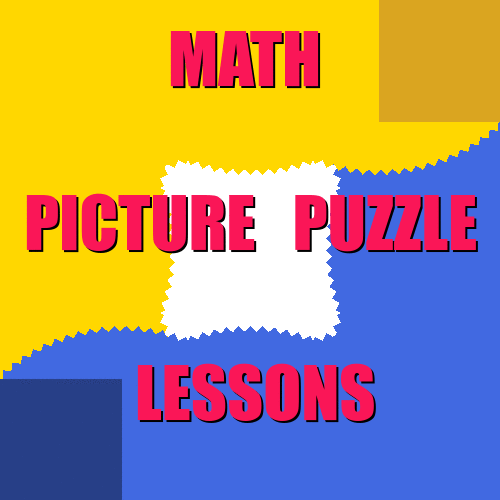Fun Cross-Number
Puzzles

New Addition and Subtraction game --- Outstanding

Teach with Touch
VG
Need Flash

COLO BY  NUMBERS  AND SIGHT  WORDS

Color sheets for you to download that will allow students to learn math. We are adding lessons that are differentiated in the first column.
Student may design their own!

Differentiation
below

listed below

Coloring on Line
Kindergarten &
listed below

Sight   Words
Kindergarten
listed below

INTERACTIVE GAMES

Click on the grade level first or just the area of study
Reading (Cause & Effect) is really good!

Balloons A 0-10 Balloons Coloring On line (1) Balloons  A-1
Balloons B 10-20 Baseball Coloring On line(2) Balloons  B-2
Ice Cream C 20-30 Butterflies Coloring On line (3) Ice Cream C-3
Ice Cream D 30-40 Cars Coloring On Line (4) Ice Cream D-4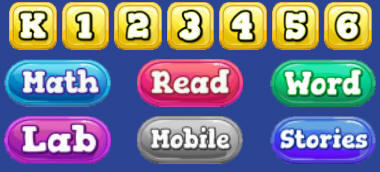Butterflies E 40-50 Ice  Cream This is Sand
Balloons F 20 - 200 Christmas -- Santa

Students may create their own lesson Blank Lesson  PDF
K - 1
Counting Dots   K - 1 A. Lesson Addition  problems counting using Dots 0 - 3    4 Minutes long
Addition    K - 1 B. Lesson Addition  problems counting using Dots 0 - 4    4 Minutes long
"   K - 1 1. Lesson Addition  problems counting using Dots 0 - 5    3 Minutes long
"   K - 1 2. Lesson Addition  problems counting using Dots 0 - 6   3 Minutes long
"   K - 1 3. Lesson Addition  problems counting using Dots 1 - 9    5 Minutes long
Subtraction   K - 1 A. Lesson Subtraction  problems counting using Dots 0 - 3    3 Minutes long
"   K - 1 B. Lesson Subtraction  problems counting using Dots 0 - 3    3 Minutes long
"   K - 1 1. Lesson Subtraction  problems counting using Dots 0 - 4   3 Minutes long
"   K - 1 2. Lesson Subtraction  problems counting using Dots 0 - 6    3 Minutes long
"   K - 1 3. Lesson Subtraction  problems counting using Dots 1 - 9    4 Minutes long
Toy Theater  Many different ideas --  Math, Reading, Art, Puzzle, Game, Classic  (This is a must see)

Play and learn the Alphabet - Numbers - Shapes - Words - Colors
(English-Spanish-French-German)

 1.  Coloring on Line 5. Alphabet 13. Star Fall 17. Common Core Math 2.  Jacobs Ladder 6. Alpha Fridge 14. Story Line 18. Elementary Subjects 3.  This Is Sand 7. Alphabetical 15. Traditional 19. Teachers Tool Box 4.  Stories 8. Word Bingo 16. Word Games 20. Flipped Classroom

MATH PUZZLES
SUBTRACTION   K - 1 1. Lesson Subtraction Math Puzzle  2 min.

Coded Message for Addition & Subtraction     1. Lesson - Addition Blank Lesson Doc
Lesson
PDF

1. Lesson - Subtraction

Numbers  with video  PDF
Subtraction  with video  PDF

SUDOKU - Puzzle   K - 1 1. Lesson Students must solve number logic puzzle
Video - How It Works   K - 1  Video 2. Lesson  Video Video lesson Students must solve number logic puzzle
K - 1 3. Lesson Students must solve number logic puzzle
K - 1 4. Lesson Students must solve number logic puzzle

PUZZLE ADD & SUB   K 1. Lesson SIMPLE ADDITION & SUBTRACTION  VIDEO  4:15 Min.
K 2. Lesson SIMPLE ADDITION & SUBTRACTION  VIDEO 4:13 Min

3-D MATH CUBED - Addition and Subtraction

1 1. Lesson Addition numbers 1 - 10
1 2. Lesson Addition numbers 10 - 20
1 3. Lesson Addition numbers 10 - 20 - 30 - 40
1 4. Lesson Addition numbers 100 - 1000

1 1. Lesson Subtraction numbers 1 - 10
1 2. Lesson Subtraction numbers 20
1 3. Lesson Subtraction numbers 30

Cross-Number Puzzle   K - 1 1E Lesson Puzzle Addition  VIDEO
K - 1 1EA Lesson Puzzle Addition  VIDEO
K - 1 1EA Lesson Puzzle Addition & Subtraction  VIDEO
K - 1 1G Lesson Puzzle Addition  VIDEO
1 1K Lesson Puzzle Addition & Subtraction  VIDEO

Telling Time   K - 1 1. Lesson This is a very simple lesson to determine the Hour Video

Money   1 E-1. Lesson Explains Coins relationships 1 - 5 - 10 - 25 cents  Easy
1 E-2. Lesson Explains Coins relationships 1 - 5 - 10 - 25 cents  Easy

Greater Than  Less Than   K 1. Lesson VIDEO  Video lessons for younger students circle the correct Objects
K 2. Lesson VIDEO   Video lessons for younger students circle the correct Objects
K 3. Lesson VIDEO   Video lessons for younger students circle the correct Number

Geography   K 1. Lesson VIDEO  Spatial Relationship
Spatial Relationship

LANGUAGE ARTS

Creating Your Story (CLICK HERE) Power Point to down load with stories Directions To create your stories, you are provided with pictures and an area to write about, the following areas are provided for you:  You may duplicate a slide if more room is needed.  You may printout all slides as a PDF file or if you wish one slide as jpg.
1) Home 2) Classroom 3)Fantasy 4)Halloween 5) Thanksgiving 6) Christmas
Video

Language Arts   K - 1 1. Lesson VIDEO Sequence in Stories PENNY SKATING  3:26
K - 1 2. Lesson VIDEO Sequence in Stories Connie and Puffy 3:43
K - 1 3. Lesson Reading Comprehension - PDF

K

Play and learn the Alphabet - Numbers - Shapes - Words - Colors
(English-Spanish-French-German)

Creating Coded Messages
Students may create their own coded messages from the blank sheet to the far right.
Lesson 1
Lesson 2
Blank sheet for students to create their own

Sight Words List
Best to use Explore for Flash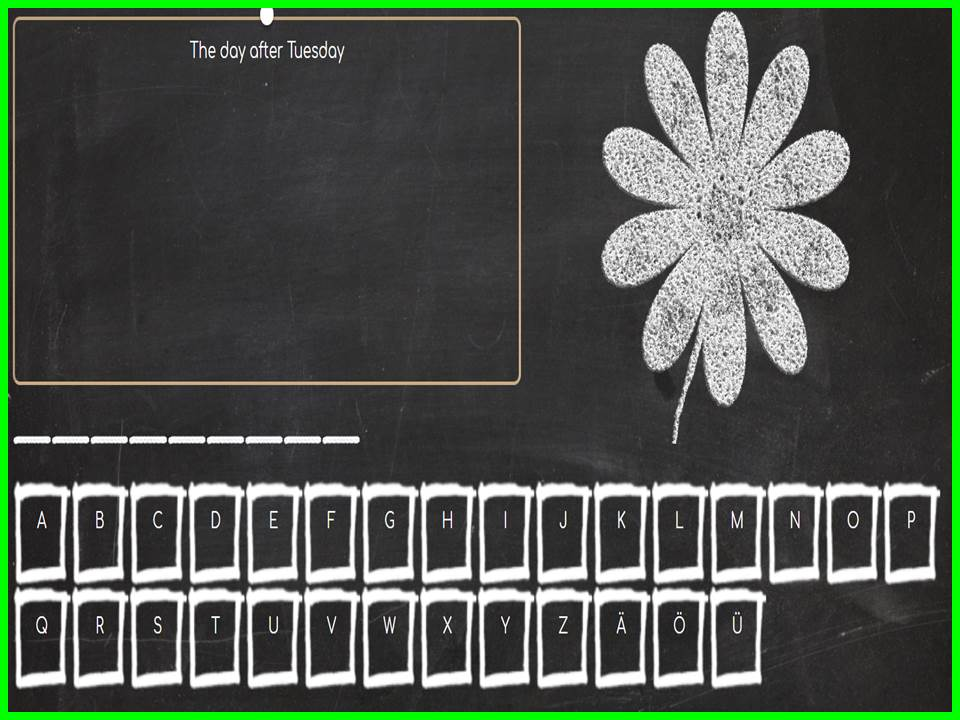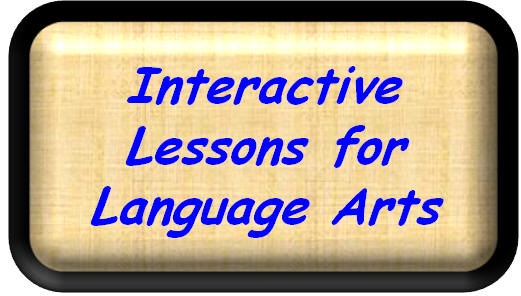Hangman

Days of the Week

Kindergarten Lessons & Worksheets

Lesson 1

Fun concept for learning spelling words.

K - 1 1. Lesson
2. Lesson

3. Lesson
4.
Lesson
5. Lesson
6. Lesson
7. Lesson
8. Lesson
9. Lesson
10. Lesson
11. Lesson
12
. Lesson
13
. Lesson
14. Lesson
15. Lesson
16. Lesson
17. Lesson
18. Lesson
19. Lesson
20. Lesson

Blank Doc  -- Blank PDF
(Students create their own coded messages with images)
Students will look at the image and spell the name from the coded message.  They may also draw the image

Sight Words   K 1. Lessons Video find sight words in a sentence.
K 2. Lessons Video find sight words in a sentence.
1 3. Lessons Video find sight words in a sentence.
K 4. Lessons Video find sight words in a sentence.
Locate words in a box.  Answer are located upside down on same sheet
KINDERGARTEN 1ST  GRADE Lessons in Morse Code
Word Search   K 1. Lesson 1 1. Compass 1. Lesson 1 8. Lesson 8 1. Lesson
K 2. Lesson 2    Answers 2. Lesson 2 9. Lesson 9 2. Lesson
K 3. Lesson 3 2. Compass 3. Lesson 3 10. Lesson10
K 4. Lesson 4      Answers 4. Lesson 4 11. Lesson11
K 5. Lesson 5   5. Lesson 5 12. Lesson12
K 6. Lesson 6   6. Lesson 6
K 7. Lesson 7 7. Lesson 7

Morse Code Trainer
(Best open up in Internet Explorer)

STORY MAKER   1 Instructional Video This video will provide you with directions on how to use this program.
PROGRAM     This is the link to the web page for these lessons.
1 1. Lesson Video showing how to create a story with some ideas.  4:15
1 Zimmer Twins Movie Maker website.  You must sign in to save cost Money \$ or just create and delete

SOCIAL STUDIES

SOCIAL STUDIES   K - 1 Teachers Toolbox Webpage

CREATING A DRAWING USING EXCEL SIMPLE AND EASY  by Brandon Pike
1. Open up Excel  from the link below.  When open at the top click on Enable Editing.
2. To place a color in the cell just click on the cell you want the color to appear (UPPER OR LOWER CASE) and type the letter of the colors listed to on the left.  r for red -- g for green bo for brown  --  bb for Black and so on

Video on how to transferring files to Google sheets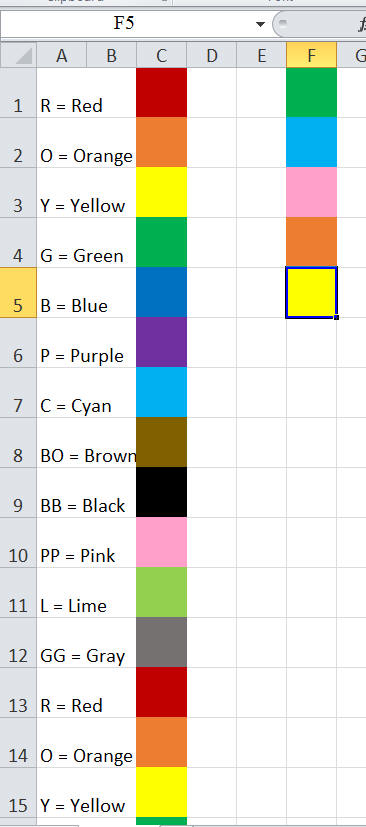1.     SMALL AREA: will fit on a one sheet of paper

a.      If your coloring is in a small area on the spreadsheet you only need to go to file then print.

2.     LARGER AREA:  will fit on more than one sheet of paper

a.      PAGE LAYOUT AT TOP MENU

b.     You may adjust to a percentage or

c.      Click FIT TO 1  BY 1